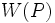# Conjugacy functor whose normalizer generates whole group with p'-core

This article defines a property that can be evaluated for a conjugacy functor on a finite group. |View all such properties

## Definition

Suppose$G$ is a group,$p$ is a prime number, and$W$ is a conjugacy functor for$G$. We say that$W$ is a conjugacy functor whose normalizer generates whole group with p'-core if it satisfies the following equivalent conditions for one (and hence every)$p$-Sylow subgroup$P$ of$G$:

1.$O_{p'}(G)N_G(W(P)) = G$
2. The image of$W(P)$ in the quotient$G/O_{p'}(G)$ is a normal subgroup of$G/O_{p'}(G)$.

## Relation with other properties

### Stronger properties

Property Meaning Proof of implication Proof of strictness (reverse implication failure) Intermediate notions
conjugacy functor that gives a normal subgroup

### Weaker properties

Property Meaning Proof of implication Proof of strictness (reverse implication failure) Intermediate notions
Conjugacy functor that controls fusion Conjugacy functor whose normalizer generates whole group with p'-core controls fusion